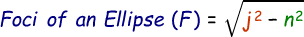# Ellipse Foci Calculator

Foci of an ellipce also known as the focus point of an ellipse lie in the center of the longest axis that is equally spaced.

Formula to calculate ellipse foci is given below:where,
F = Distance from each focus to centerĀ
j = Major axis radius
n = Minor axis radius

In the below online ellipse foci calculator, enter the radius of major axis and minor axis and then click calculate to find the answer.

 Radius of Major Axis (j): Radius of Minor Axis (n): Ellipse Foci:

Related Calculator: Average Value of a Function Calculator

Latest Calculator Release

Average Acceleration Calculator

Average acceleration is the object's change in speed for a specific given time period. ...

Free Fall Calculator

When an object falls into the ground due to planet's own gravitational force is known a...

Torque Calculator

Torque is nothing but a rotational force. In other words, the amount of force applied t...

Average Force Calculator

Average force can be explained as the amount of force exerted by the body moving at giv...

Angular Displacement Calculator

Angular displacement is the angle at which an object moves on a circular path. It is de...